We are going to study Batch Norm, Weight Norm, Layer Norm, Instance Norm, Group Norm, Batch-Instance Norm, Switchable Norm{#591f}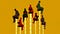Let's start with the question,{#d0f6}

Normalization has always been an active area of research in deep learning. Normalization techniques can decrease your model's training time by a huge factor. Let me state some of the benefits of using Normalization.{#5fbf}

1. It normalizes each feature so that they maintains the contribution of every feature, as some feature has higher numerical value than others. This way our network can be unbiased(to higher value features).{#5ea7}
{#5ea7}
2. It reduces Internal Covariate Shift . It is the change in the distribution of network activations due to the change in network parameters during training. To improve the training, we seek to reduce the internal covariate shift.{#af62}
{#af62}
3. In this paper, authors claims that Batch Norm makes loss surface smoother(i.e. it bounds the magnitude of the gradients much more tightly).{#ceb0}
{#ceb0}
4. It makes the Optimization faster because normalization doesn't allow weights to explode all over the place and restricts them to a certain range.{#b5c7}
{#b5c7}
5. An unintended benefit of Normalization is that it helps network in Regularization(only slightly, not significantly).{#02db}
{#02db}

From above, we can conclude that getting Normalization right can be a crucial factor in getting your model to train effectively, but this isn't as easy as it sounds. Let me support this by certain questions.{#35dc}

1. How Normalization layers behave in Distributed training ?{#90a4}
{#90a4}
2. Which Normalization technique should you use for your task like CNN, RNN, style transfer etc ?{#c737}
{#c737}
3. What happens when you change the batch size of dataset in your training ?{#81be}
{#81be}
4. Which norm technique would be the best trade-off for computation and accuracy for your network ?{#6c84}
{#6c84}

To answer these questions, Let's dive into details of each normalization technique one by one.{#b506}

Batch normalization is a method that normalizes activations in a network across the mini-batch of definite size. For each feature, batch normalization computes the mean and variance of that feature in the mini-batch. It then subtracts the mean and divides the feature by its mini-batch standard deviation.{#3107}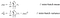this image is taken from https://arxiv.org/pdf/1502.03167.pdf'

But wait, what if increasing the magnitude of the weights made the network perform better?{#b01d}

To solve this issue, we can add γ and β as scale and shift learn-able parameters respectively. This all can be summarized as:{#436e}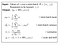ϵ is the stability constant in the equation.

## Problems associated with Batch Normalization : {#e257}

1. Variable Batch Size → If batch size is of 1, then variance would be 0 which doesn't allow batch norm to work. Furthermore, if we have small mini-batch size then it becomes too noisy and training might affect. There would also be a problem in distributed training . As, if you are computing in different machines then you have to take same batch size because otherwise γ and β will be different for different systems.{#2096}
{#2096}
2. Recurrent Neural Network → In an RNN, the recurrent activations of each time-step will have a different story to tell(i.e. statistics). This means that we have to fit a separate batch norm layer for each time-step. This makes the model more complicated and space consuming because it forces us to store the statistics for each time-step during training.{#4782}
{#4782}

Batch norm alternatives(or better norm s) are discussed below in details but if you only interested in very short description(or revision just by look at an image) look at this :{#1d8e}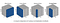This image is taken from https://arxiv.org/pdf/1803.08494.pdf

Wait, why don't we normalize weights of a layer instead of normalizing the activations directly. Well, Weight Normalization does exactly that.{#168f}

Weight normalization reparameterizes the weights ( ω ) as :{#92cf}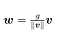It separates the weight vector from its direction, this has a similar effect as in batch normalization with variance. The only difference is in variation instead of direction.{#b12c}

As for the mean, authors of this paper cleverly combine mean-only batch normalization and weight normalization to get the desired output even in small mini-batches. It means that they subtract out the mean of the minibatch but do not divide by the variance. Finally, they use weight normalization instead of dividing by variance.{#3b01}

Note: Mean is less noisy as compared to variance(which above makes mean a good choice over variance) due to thelaw of large numbers . {#ea19}

The paper shows that weight normalization combined with mean-only batch normalization achieves the best results on CIFAR-10.{#b097}

Layer normalization normalizes input across the features instead of normalizing input features across the batch dimension in batch normalization.{#f031}

A mini-batch consists of multiple examples with the same number of features. Mini-batches are matrices(or tensors) where one axis corresponds to the batch and the other axis(or axes) correspond to the feature dimensions.{#5d92}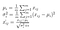i represents batch and j represents features. xᵢ,ⱼ is the i,j-th element of the input data.

The authors of the paper claims that layer normalization performs better than batch norm in case of RNN s.{#acbb}

Layer normalization and instance normalization is very similar to each other but the difference between them is that instance normalization normalizes across each channel in each training example instead of normalizing across input features in an training example. Unlike batch normalization, the instance normalization layer is applied at test time as well(due to non-dependency of mini-batch).{#b494}This image is taken from https://arxiv.org/pdf/1607.08022.pdf

Here, x ∈ ℝ T ×C×W×H be an input tensor containing a batch of T images. Let x ₜᵢⱼₖ denote its tijk-th element, where k and j span spatial dimensions( H eight and W idth of the image), i is the feature channel (color channel if the input is an RGB image), and t is the index of the image in the batch.{#ec58}

This technique is originally devised for style transfer , the problem instance normalization tries to address is that the network should be agnostic to the contrast of the original image.{#66d3}

As the name suggests, Group Normalization normalizes over group of channels for each training examples. We can say that, Group Norm is in between Instance Norm and Layer Norm.{#2835}

∵ When we put all the channels into a single group, group normalization becomes Layer normalization. And, when we put each channel into different groups it becomes Instance normalization. {#f54e}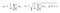Sᵢ is defined below.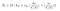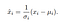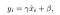Here, x is the feature computed by a layer, and i is an index. In the case of 2D images, i = ( i N , i C , i H, i W ) is a 4D vector indexing the features in (N, C, H, W) order, where N is the batch axis, C is the channel axis, and H and W are the spatial height and width axes. G is the number of groups, which is a pre-defined hyper-parameter. C/G is the number of channels per group. ⌊.⌋ is the floor operation, and " ⌊kC/(C/G)⌋= ⌊iC/(C/G)⌋ " means that the indexes i and k are in the same group of channels, assuming each group of channels are stored in a sequential order along the C axis. GN computes µ and σ along the (H, W) axes and along a group of C/G channels.{#a539}

The problem with Instance normalization is that it completely erases style information. Though, this has its own merits(such as in style transfer) it can be problematic in those conditions where contrast matters(like in weather classification, brightness of the sky matters). Batch-instance normalization attempts to deal with this by learning how much style information should be used for each channel( C ).{#750c}

Batch-Instance Normalization is just an interpolation between batch norm and instance norm.{#0820}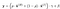the value of ρ is in between 0 and 1.

The interesting aspect of batch-instance normalization is that the balancing parameter ρ is learned through gradient descent.{#0eb0}

From batch-instance normalization, we can conclude that models could learn to adaptively use different normalization methods using gradient descent. {#37fa}

Understanding from above, a question may arise.{#a707}

## Can we switch the normalization technique whenever needed ? {#9c1b}

The answer would be Yes. Following technique does exactly that.{#9f4c}

This paper proposed switchable normalization, a method that uses a weighted average of different mean and variance statistics from batch normalization, instance normalization, and layer normalization.{#72c4}

The authors showed that switch normalization could potentially outperform batch normalization on tasks such as image classification and object detection.{#ec51}

The paper showed that the instance normalization were used more often in earlier layers, batch normalization was preferred in the middle and layer normalization being used in the last more often. Smaller batch sizes lead to a preference towards layer normalization and instance normalization.{#de24}

(未经同意，请勿转载)